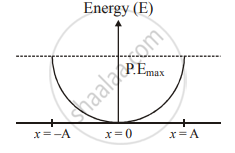# Obtain an Expression for Potential Energy of a Particle Performing Simple Harmonic Motion. Hence Evaluate the Potential Energy - Physics

Numerical

Obtain an expression for potential energy of a particle performing simple harmonic motion. Hence evaluate the potential energy

1. at mean position and
2. at extreme position.

#### Solution

An oscillating particle possess both types of energies : Potential as well as kinetic. It possesses potential energy on account of its displacement from the equilibrium position.
Potential energy : Consider a particle of mass m executing S.H.M. Let x be its displacement from the equilibrium position at any instant t. Since in S.H.M. the force F acting upon the particle is proportional to and opposite to the displacement x, we have
F = -kx
Where, the constant k gives the force per unit displacement . The force can also be express in terms of potential energy (P.E) of the particle as :
F = -(dw)/(dx)
Thus,  (dw)/(dx) = kx
On integrating, we get
P.E = 1/2 kx^2 + C
Where  is a constant. If we assume the potential energy zero in the equilibrium position, i.e.,
If P.E = 0 at x = 0, then C = 0
Therefore, P.E = 1/2kx^2
"P"."E" = 1/2mw^2x^2
Hence from here it is clear that the potential energy of a particle doing S.H.M. is directly proportional to the square of the displacement (P.E.alpha x^2)
(a) Potential energy at mean position : At mean position, velocity of a particle executing S.H.M. is maximum and displacement is minimum i.e., x =
P.E = 1/2 mw^2x^2 = 0
(b) Potential energy at extreme position : At extreme position, velocity of a particle executing S.H.M. is minimum and displacement is maximum, i.e., x =
P.E = 1/2 ka^2 = 1/2mw^2a^2Concept: K.E.(Kinetic Energy) and P.E.(Potential Energy) in S.H.M.
Is there an error in this question or solution?

Share